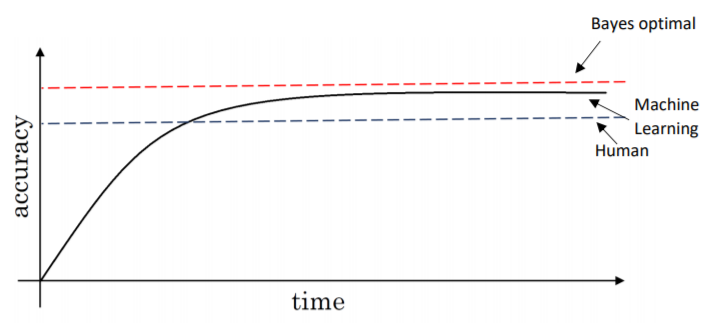# What is the maximum accuracy that a Machine Learning model can achieve?

Original article was published by Uttaran Tribedi on Artificial Intelligence on MediumBut does this higher value of accuracy necessarily mean a better model? The short answer is no. The long answer depends on multiple factors. So let’s break it down into some possible scenarios:

Firstly, if we are talking about a single parameter evaluation metric for the entire dataset then we have to judge on the given problem at hand and the nature o the dataset. After the analysis, it may happen that Accuracy is the chosen parameter (for example when we have a symmetric dataset and the values of false positives and false negatives are almost the same) or something else like Precision, Recall, etc.

The second case is that when we want our single parameter to be a reflection of more than a single indicator. The best example of this is the F1 score. It is used when the user wants to take into account both the values of Precision and Recall (most likely when the class distribution is uneven or the cost of false positives or false negatives are very different from each other). F1 score is calculated by taking the weighted average or in other words the Harmonic Mean of Precision and Recall i.e

F1 score = (2* Precision * Recall)/(Precision + Recall)

The third case can be that we need to take into account multiple parameters simultaneously and unlike the previous case, say we are not able to condense it into a single value. So what do we do then? The approach is to divide the parameters that we want to include into two groups- the optimising and the satisficing metrics. The optimising metric will be the single parameter whose value we want to optimise to the maximum extent possible. The satisficing metrics will involve all the other parameters which are required to stay above a certain threshold. Each of the satisficing metrics is expected to have their own threshold values. So long as they are above their expected threshold, it doesn’t really matter how much their magnitudes are. So once the satisficing metrics are taken care of, we can focus our entire attention on getting the best possible value of our optimising metric, which will lead us to get the best results for our task.